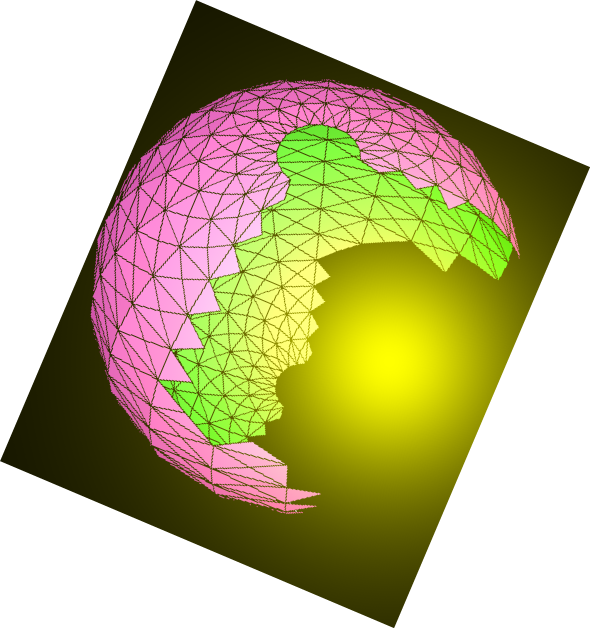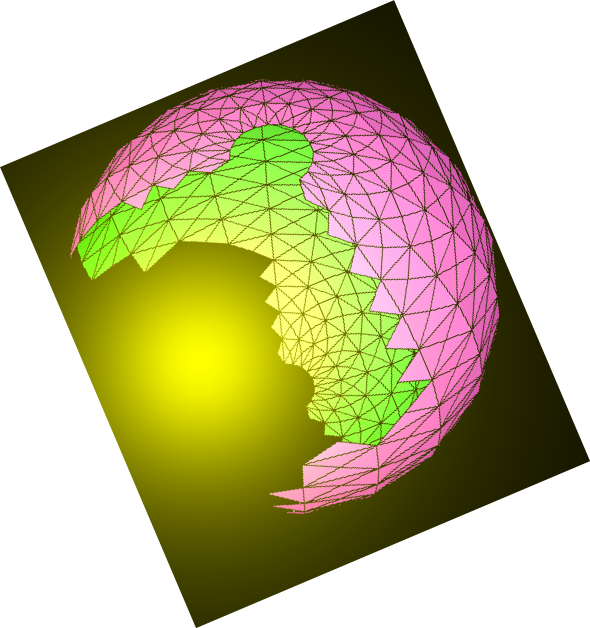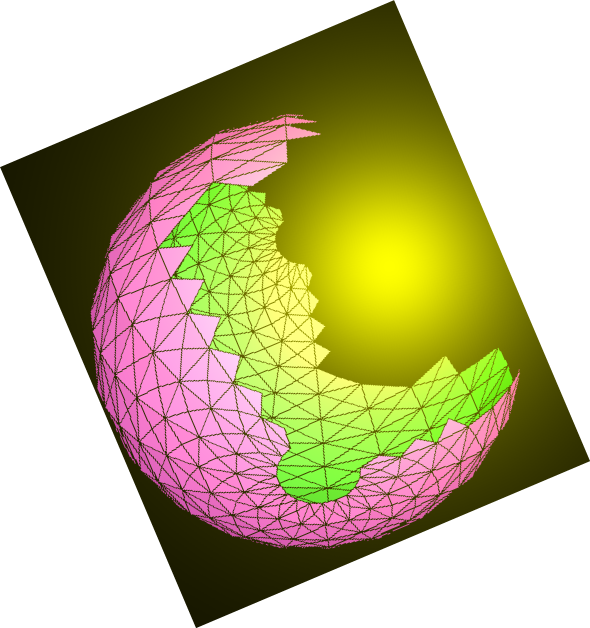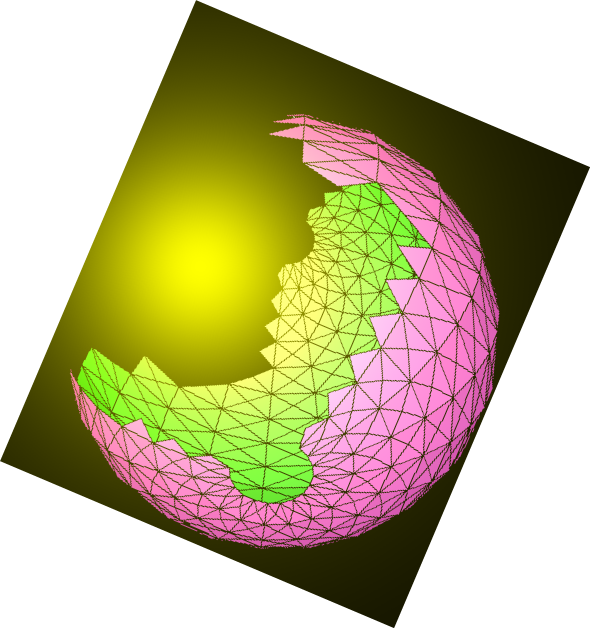# N.S.B. Cosmic Center### Invariant Field Theory by Nazir S. Baaklini

Back to DCSC Books

#### Contents

Introduction: Field theory in four dimensions. Fermi-Bose symmetry. Extra dimensions.

Theory of Fundamental Fields

Action Principles: Spinless scalars. Spin-half spinors. Spin-one vectors. Spin-3/2 fields. Spin-2 tensors. Reflective symmetries.

Algebraic Gauge Principles: Non-commutative gauge formalism. Simple algebraic gauge systems. Scalar self-interactions. Yukawa couplings.

Symmetry Breaking: General theory. Orthogonal gauge theory. Unitary gauge theory. Symplectic gauge theory. Exceptional gauge theory.

Supersymmetric Field Theory

Fundamental Supermultiplets: Superfield calculus. Scalar supermultiplet. Other supermultiplets.

Supersymmetric Gauge Theory: Superfield gauge formalism. Instructive problems. Symmetry breaking. Local Supersymmetry.

Higher-Dimensional Field Theory

Higher-Dimensional Action Principles: The approach to extra dimensions. Unified gauge theories. Spinor fields. Internal symmetries. Instructive examples.

Extended and Higher-Dimensional Supersymmetries: N=2 extended supermultiplets. N=4 extended supermultiplets. N=8 extended supermultiplet. Six and ten-dimensional supersymmetric gauge theory. Supermultiplet in eleven dimensions.

Appendix: Theory of Currents

Bibliography

Back to DCSC BooksHome
N.S.B. Cosmic Center by Nazir S. Baaklini